# Numerical Solutions of the One Dimensional Navier Stokes Equation¶

## Introduction ¶

Computational Fluid Dynamics (or CFD for short) remains a difficult field to get into. Despite a wealth of open source software, the main being OpenFOAM (https://openfoam.org/ or is it https://openfoam.com), there remains a massive barrier to new students and even a lack of resource for senior academics to learn and understand the basics.

This is, in part, due to the semi-commerical nature of fluid dynamics. By keeping barriers high, consultancy money can be made and forums like CFD Online (https://www.cfd-online.com/Forums/) seem to be populated by lost academic searching for simple answers.

Arguably, no one should be writing their own CFD solver. In the interest of software best practice (https://www.software.ac.uk/), we should be contributing to one of the numerous open-source project in the form on tests, bug-fixes and features. However, we need to understand what these codes are doing in order to have a chance of using them. This is where minimal code examples come in.

Inspired by the excellent introduction to fluid dynamics given by Professor Barba of George Washington University http://lorenabarba.com/blog/cfd-python-12-steps-to-navier-stokes/ and the amazing minimal MATLAB Navier Stokes solver provided by Gretar Tryggvason of Johns Hopkins University https://www3.nd.edu/~gtryggva/MultiphaseDNS/, this tutorial aims to provide a complete example of a fluid solver in one dimension.

Although useless for solving real world problem, it provides a minimal insight into the numerics, details of how we solve for the pressure and how to set the most common boundary conditions. Using this minimal solver, it is easy to test changes and see how they effect the solutions. Used together with a "real" CFD package, this can provide a very effective way of understanding and debugging CFD applications. They also provide excellent starting points for testing more complex applications, such as novel numerical methods, multi-phase flows or even coupled simulation (http://www.cpl-library.org).

To run these examples, either download jupyter (https://jupyter.org/install) or you can use the free trial version in your web browser (https://jupyter.org/try) and click Python.

## The Navier Stokes Equation ¶

The one dimensional version of the Navier Stokes Equation looks as follows,

\begin{equation} \underbrace{\frac{\partial u}{\partial t}}_{\textrm{Unsteady Term}} +\underbrace{u \frac{\partial u}{\partial x}}_{\textrm{Advection Term}} = - \frac{1}{\rho}\underbrace{\frac{\partial P}{\partial x}}_{\textrm{Pressure Term}} + \underbrace{\frac{\mu}{\rho} \frac{\partial^2 u}{\partial x^2}}_{\textrm{Diffusion Term}} \end{equation}

Note we have one equation but two unknowns (pressure $P$ and velocity $u$). For simplicity, we will set density to unity $\rho=1$. To solve this we need another equation, which we get by assuming incompressible mass continuity equation (other assumptions are available),

\begin{equation} \frac{\partial u}{\partial x} = 0 \end{equation}

The fractional step or Chorin (https://en.wikipedia.org/wiki/Projection_method_%28fluid_dynamics%29) method works by making a pressure free initial guess and the correcting for the pressure using the condition of continuity. So, we start by taking the Navier Stokes equation without pressure and solving this numerically,

\begin{equation} \underbrace{\frac{\partial u}{\partial t}}_{\textrm{Unsteady Term}} +\underbrace{u \frac{\partial u}{\partial x}}_{\textrm{Advection Term}} = \underbrace{\mu \frac{\partial^2 u}{\partial x^2}}_{\textrm{Diffusion Term}} \end{equation}

Then substituing back using the correction based on the mass continity to get the pressure in a field which satisfies contiuity. We will come back to this after looking at the numerical solution to these three terms.

## Numerical Methods ¶

The formal definition of a derivative is,

\begin{equation} \frac{\partial u}{\partial t} = \lim_{\Delta x \to 0} \frac{u(x+\Delta x) - u(x)}{ \Delta t} \end{equation}

Numerical methods mean we approximate derivatives by making $\Delta x$ arbitarily small,

\begin{equation} \frac{\partial u}{\partial t} \approx \frac{u(x+\Delta x) - u(x)}{ \Delta t} \end{equation}

Introducting the superscript notation to define that a point $\Delta x$ away from $x$ is a different node or cell, we can define $u(x) \equiv x_i$ and $u(x+\Delta x) \equiv x_{i+1}$. Similarly for time, superscript notation is employed $u(t) = u^t$ and $u(t+\Delta t) = u^{t+1}$.

Programatically, let's start by importing modules and defining some constants.

In :
import numpy as np
import matplotlib.pyplot as plt
#Constants, number of cell, timestep and cell size
Nx = 10
dt = 0.001
dx = 0.1
u = np.zeros(Nx)


Note that the value of $u = u(x,t) \equiv u_i^t$ is defined at every point in space and every time. So, in a discrete world, this means it needs to be define at a number of cell values in space ($Nx=10$ here, with each cell denoted by subscript $i$). We have allocated a numpy array of size Nx to contant the value of velocity at each point in space. The values at each time (denoted by superscript $t$) is then obtained by replacing the previous value in the variable $u$.

## Unsteady Term ¶

So, out first step is to discretise both sides of the equation, Taking the left hand side first

\begin{equation} \frac{\partial u}{\partial t} \approx \frac{u_i^{t+1} - u_i^t}{\Delta t} \end{equation}

Let's say we want to solve the time evolution is a constant,

\begin{equation} \frac{\partial u}{\partial t} = a \end{equation}

We would discretise it to,

\begin{equation} \frac{u_i^{t+1} - u_i^t}{\Delta t} = a \end{equation}

And rearrange so the time evolution is,

\begin{equation} u_i^{t+1} = - u_i^t + \Delta t a \end{equation}

Computationally, this looks as follows (note we loop over all cells $Nx$),

In :
u = np.zeros(Nx)
a = 1.0
for i in range(Nx):
u[i] = u[i] + dt * a
print(i, u[i])

(0, 0.001)
(1, 0.001)
(2, 0.001)
(3, 0.001)
(4, 0.001)
(5, 0.001)
(6, 0.001)
(7, 0.001)
(8, 0.001)
(9, 0.001)


Remember in computing, the equals means to asign what is on the left to the value on the right. If we run this again, we get $u^{t+2}$

In :
for i in range(Nx):
u[i] = u[i] + dt * a
print(i, u[i])

(0, 0.002)
(1, 0.002)
(2, 0.002)
(3, 0.002)
(4, 0.002)
(5, 0.002)
(6, 0.002)
(7, 0.002)
(8, 0.002)
(9, 0.002)


and so on, each time replacing the $Nx$ cell values with the new timestep. Note that we need to set a boundary condition here in order to copnverge to a solution.

TO DO:

• Straight line example, B.C. is intercept, a is the graident
• Compare to exact integral

## Advection Term ¶

Solve the time-evolving advection equation:

\begin{equation} \frac{\partial u}{\partial t} = -u \frac{\partial u}{\partial x} \end{equation}

So, out first step is to discretise both sides of the equation, Taking the left hand side first

\begin{equation} \frac{\partial u}{\partial t} \approx \frac{u_i^{t+1} - u_i^t}{\Delta t} \end{equation}

and then for the right hand side, we use a backward difference scheme (for reasons we will discuss soon),

\begin{equation} u \frac{\partial u}{\partial x} \approx u_i^t \frac{ u_{i}^{t} - u_{i-1}^t}{\Delta x} \end{equation}

which means we have

\begin{equation} \frac{u_i^{t+1} - u_i^t}{dt} = -u_i^t \frac{u_{i}^{t} - u_{i-1}^t}{\Delta x} \end{equation}

and we rearrange to get the velocity at the next time t+1.

\begin{equation} u_i^{t+1}= u_i^t - \Delta t \; u_i^t \frac{u_{i}^{t} - u_{i-1}^t}{\Delta x} \end{equation}

which we can write as code in the following way

In :
#Setup velocity to one everywhere and 2 in a region
u = np.ones(Nx)
u[3:6] = 2.

#Plot initial and range of values
plt.plot(u)
for n in range(200):
for i in range(Nx-1):
u[i] = u[i] - dt*u[i] * (u[i] - u[i-1])/dx

u = u[-2]
u[-1] = u

if n%20 == 0:
plt.plot(u)
plt.show()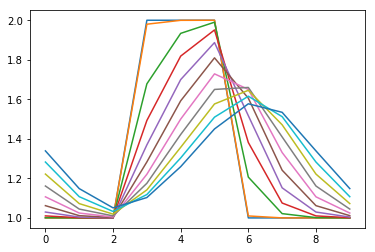This is known as upwinding, the velocity is always used down from the direction of flow. If we don't do this, we observe oscillations. Consider a forward difference

\begin{equation} u_i^t \frac{u_{i+1}^{t} - u_i^t}{\Delta x} \end{equation}

In :
#Setup velocity to one everywhere and 2 in a region
u = np.ones(Nx)
u[3:6] = 2.

#Plot initial and range of values
plt.plot(u)
for n in range(20):
for i in range(Nx-1):
u[i] = u[i] - dt*u[i]*(u[i+1]-u[i])/dx

u = u[-2]
u[-1] = u

plt.plot(u)
plt.show()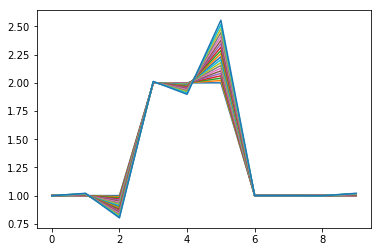Notice we see Gibbs phenomena like oscilations here, which grow as the simulation proceeds.

### Oscillations ¶

This is well known to happen for second order schemes, here we use a central difference scheme.

In :
#Setup velocity to one everywhere and 2 in a region
u = np.ones(Nx)
u[3:6] = 2.

#Plot initial and range of values
plt.plot(u)
for n in range(20):
for i in range(Nx-1):
u[i] = u[i] - dt*u[i] * (u[i+1] - u[i-1])/(2.*dx)

u = u[-2]
u[-1] = u

plt.plot(u)
plt.show()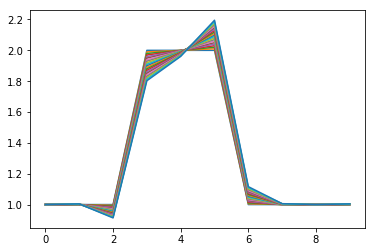## Diffusion Term ¶

Next we look at the one dimensional form of the unsteady diffusion equation, \begin{equation} \frac{\partial u}{\partial t} = \nu \frac{\partial^2 u}{\partial x^2} \end{equation} We discretise the unsteady term on the left hand side as before. The second order diffusive term on the right hand side can be discretised as follows, \begin{equation} \frac{\partial^2 u}{\partial x^2} \approx \frac{ u_{i+1}^{t} - 2 u_{i+1}^{t} + u_{i-1}^t}{(\Delta x)^2} \end{equation} where the process of approximation is applied twice, easiest to see with a substitution. \begin{equation} \frac{\partial^2 u}{\partial x^2} = \frac{\partial g}{\partial x} \approx \frac{ g_{i+1} - g_{i}}{\Delta x} \end{equation} where $g=\frac{\partial u}{\partial x}$. We can discretise this in the same way, this time using a backward difference to make sure the second derivative ends up being symmetrical about the cell $i$ we are interested in, \begin{equation} \frac{\partial u}{\partial x} = \approx \frac{ u_{i} - u_{i-1}}{\Delta x} \end{equation} note that the indices simply add so $g_{i+1} \approx \frac{ u_{i+1} - u_{i}}{dx}$ and putting these back into, \begin{equation} \frac{\partial^2 u}{\partial x^2} \approx \frac{ g_{i+1} - g_{i}}{\Delta x} = \frac{ u_{i} - u_{i-1} - (u_{i+1} - u_{i})}{(\Delta x)^2} = \frac{ u_{i+1}^{t} - 2 u_{i+1}^{t} + u_{i-1}^t}{(\Delta x)^2} \end{equation}

In :
#Reset velocity ready for next example
u = np.zeros(Nx)
u[3:6] = 1.

#Plot initial and range of values
nu =1.0
for n in range(20):
for i in range(Nx-1):
u[i] = u[i] + dt*nu*(u[i+1]-2.*u[i]+u[i-1])/dx**2

#Periodic boundary conditions
u = u[-2]
u[-1] = u

plt.plot(u)
plt.show()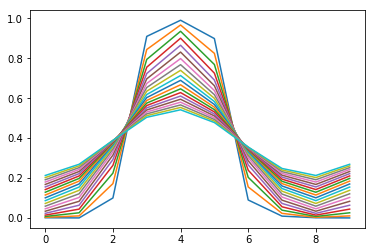## Pressure Term ¶

So, the solution of the Navier Stokes equation without pressure can be obtained from the three numerical approximations already outlined,

\begin{equation} \underbrace{\frac{\partial u}{\partial t}}_{\textrm{Unsteady Term}} +\underbrace{u \frac{\partial u}{\partial x}}_{\textrm{Advection Term}} = \underbrace{\mu \frac{\partial^2 u}{\partial x^2}}_{\textrm{Diffusion Term}} \end{equation}

Which becomes,

\begin{equation} \underbrace{ \frac{u_i^{t+*} - u_i^t}{\Delta t}}_{\textrm{Unsteady Term}} +\underbrace{u_i^t \frac{ u_{i}^{t} - u_{i-1}^t}{\Delta x}}_{\textrm{Advection Term}} = \underbrace{\mu \frac{ u_{i+1}^{t} - 2 u_{i+1}^{t} + u_{i-1}^t}{(\Delta x)^2} }_{\textrm{Diffusion Term}} \end{equation}

Note the $t+*$ denotes that this isn't the next time but how the flow would evolve without the pressure gradient. We can rearrange this to get $u_i^{t+*}$ as follows,

\begin{equation} u_i^{t+*} = u_i^t + \frac{\Delta t}{\Delta x} \left[-u_i^t \left( u_{i}^{t} - u_{i-1}^t \right) + \mu \frac{ u_{i+1}^{t} - 2 u_{i}^{t} + u_{i-1}^t}{\Delta x} \right] \end{equation} Consider, what we have actually done is split the time evolution into two parts, 1) due to advective and diffusion terms and 2) due to pressure gradient,

\begin{equation} \underbrace{\frac{u_i^{t+1} - u_i^{t+*}}{\Delta t} + \frac{u_i^{t+*} - u_i^t}{\Delta t}}_{\textrm{Unsteady Term}} = -\underbrace{u \frac{\partial u}{\partial x}}_{\textrm{Advection Term}} - \underbrace{\frac{\partial P}{\partial x}}_{\textrm{Pressure Term}} + \underbrace{\mu \frac{\partial^2 u}{\partial x^2}}_{\textrm{Diffusion Term}} \end{equation}

So to get the next timestep, $u_i^{t+1}$, we take the value which includes the advective and diffusion parts $u_i^{t+*}$ and then add the pressure term, \begin{equation} \frac{u_i^{t+1} - u_i^{t+*}}{\Delta t} = -\frac{\partial P}{\partial x} \approx -\frac{P_{i+1} - P_i}{\Delta x} \end{equation}

But we cannot solve for pressure as it is a second unknown ($u$ being the first) and we have only one equations (the 1D Navier Stokes). However, we know from continuity our velocity solution at $t+1$ must satisfy the condition that, \begin{equation} \frac{\partial u}{\partial x} \approx \frac{ u_{i}^{t+1} - u_{i-1}^{t+1}}{\Delta x} = 0 \end{equation} Note we've used a backward difference expression to keep expression symmetrical about cell $i$. We we can substitute the expression for $u_i^{t+1}$ in term of pressure and $u_i^{t+*}$, which is, \begin{equation} u_i^{t+1} = u_i^{t+*} -\Delta t \frac{P_{i+1} - P_i}{\Delta x} \end{equation} and for $u_{i+1}^{t+1}$ \begin{equation} u_{i-1}^{t+1} = u_{i-1}^{t+*} -\Delta t \frac{P_{i} - P_{i-1}}{\Delta x} \end{equation} so the continuity equation is, \begin{equation} \frac{ u_{i+1}^{t+1} - u_{i}^{t+1}}{\Delta x} = \frac{ (u_{i-1}^{t+*} -\Delta t \frac{P_{i} - P_{i-1}}{\Delta x}) - (u_i^{t+*} -\Delta t \frac{P_{i+1} - P_i}{\Delta x})}{\Delta x} = \Delta t \frac{ P_{i+1} - 2P_{i} + P_{i-1}}{(\Delta x)^2} \end{equation}

Which is Poisson equation, (https://en.wikipedia.org/wiki/Poisson's_equation) \begin{equation} \frac{\partial^2 P}{\partial x^2} = \frac{\partial u^{t+*}}{\partial x} \end{equation} assuming that velocity derivative is a constant, we can solve this by simply iterating until pressure stops changing. Note that we need to define boundary conditions for pressure

We first rearrange to get the next value of $P_i$ from the surrounding values, \begin{equation} P_{i} = \frac{1}{2} \left( P_{i+1} + P_{i-1} - \frac{\Delta x}{\Delta t } \left[ u_{i+1}^{t+*} - u_{i}^{t+*} \right]\right) \equiv \frac{1}{2} \left( P_{i+1} + P_{i-1}\right) + b \end{equation} where $b$ is defined to include all velocity components, \begin{equation} b \equiv- \frac{\Delta x}{2\Delta t } \left[ u_{i+1}^{t+*} - u_{i}^{t+*} \right] \end{equation}

In :
maxit = 100
p = np.zeros(Nx)
for it in range(maxit):
pn = p.copy()
i = np.arange(1,Nx-1)
b = -0.5*(dx)*(u[i+1]-u[i])
p[i] = 0.5*(p[i+1]+p[i-1])+b

#Pressure boundary conditions
p = p[-2]
p[-1] = p

plt.plot(p)
plt.show()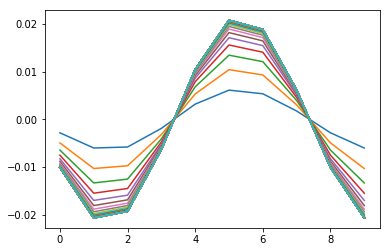Adding a converegence condition can help to reduce unneeded computations,

In :
maxit = 100
tol = 1e-4
p = np.zeros(Nx)
for it in range(maxit):
pn = p.copy()
i = np.arange(1,Nx-1)
b = -0.5*(dx)*(u[i+1]-u[i])
p[i] = 0.5*(p[i+1]+p[i-1])+b

#Pressure boundary conditions
p = p[-2]
p[-1] = p

plt.plot(p)
error = abs(pn-p).max()
if (error < tol):
print("Needed " + str(it) + " steps to get accuracy of " + str(error))
break

plt.show()

Needed 12 steps to get accuracy of 9.952561276536237e-05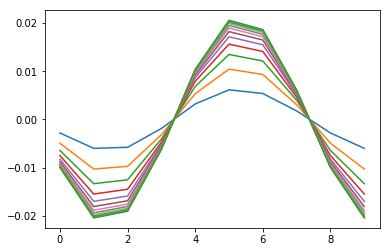Matrix Inversion

In :
# Define a tridiagonal matrix with i on the diagonal
# (times two) and i+1 and i-1 values either side
# See https://www-m16.ma.tum.de/foswiki/pub/M16/Allgemeines/StefanPossanner/Poisson1D_FD.html
A = (  np.diag(np.ones(Nx-3), -1)
- np.diag(2.*np.ones(Nx-2), 0)
+ np.diag(np.ones(Nx-3), 1))

i = np.arange(1,Nx-1)
b = -0.5*(dx)*(u[i+1]-u[i])
P = np.dot(b, np.linalg.inv(A).T)

solver_err = np.max(np.abs(A*P-b) )
print(solver_err, A)
plt.plot(P)
plt.show()

(0.030583033279878068, array([[-2.,  1.,  0.,  0.,  0.,  0.,  0.,  0.],
[ 1., -2.,  1.,  0.,  0.,  0.,  0.,  0.],
[ 0.,  1., -2.,  1.,  0.,  0.,  0.,  0.],
[ 0.,  0.,  1., -2.,  1.,  0.,  0.,  0.],
[ 0.,  0.,  0.,  1., -2.,  1.,  0.,  0.],
[ 0.,  0.,  0.,  0.,  1., -2.,  1.,  0.],
[ 0.,  0.,  0.,  0.,  0.,  1., -2.,  1.],
[ 0.,  0.,  0.,  0.,  0.,  0.,  1., -2.]]))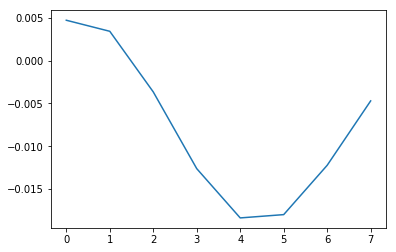Boundary Conditions

## The 1D Navier Stokes ¶

Putting all of this together, we have a one dimensional version of the Navier Stokes Equation

In :
import numpy as np
import matplotlib.pyplot as plt

#Coefficients
Lx = 2.0 #Domain size
nx = 44 #Number of cells
nt = 400    #nt is the number of timesteps
dt = 0.0025  #dt is the timestep (delta t)
maxit = 100 #Pressure solver maximum iteration
tol = 1e-4  #Pressure solver maximum error
nu = 0.01 #Viscosity
plotfreq = 100 #Output plot frequency

#Switch bits of the solver on
on = {}
on["advection"] = True
on["diffusion"] = True
on["pressure_calc"] = True
on["p_correction"] = True

#Initialise fields
dx = Lx/(nx-1.) #Cell size
u = np.zeros(nx+1)
p = np.zeros(nx)

#Set initial condition u = 1.0 between 0.5 and 1
start = int(0.25*Lx/dx)
end = int(0.5*Lx/dx + 1)
u[start:end] = 1.0

#iterate through time
for n in range(nt):

#Advection and diffusion to project pressure free velocity
i = np.arange(1,nx)
un = u.copy()
if on["advection"]:
u[i] -= dt*(un[i]*(un[i]-un[i-1])/(2.*dx))
if on["diffusion"]:
u[i] += dt*nu*(un[i+1]-2.*un[i]+un[i-1])/dx**2

#Get Pressure using incompressible continity eqn
if on["pressure_calc"]:
for it in range(maxit):
pn = p.copy()
i = np.arange(1,nx-1)
b = -0.5*(dx)*(u[i+1]-u[i-1])
p[i] = 0.5*(p[i+1]+p[i-1])+b

#Pressure boundary conditions
p = p[-2]
p[-1] = p

error = abs(pn-p).max()
if (error < tol):
#print("p correct = ", it, error)
break

#Correct pressure free field
if on["p_correction"]:
i = np.arange(1,nx-1)
u[i] -= 2.*dt*(p[i+1]-p[i])/dx

#Velocity boundary conditions
u = u[-2]
u[-1] = u

#Plot
if n%plotfreq == 0:
plt.plot(u, 'r-', label="Velocity")
plt.plot(p, 'b-', label="Pressure")
plt.legend()
plt.show()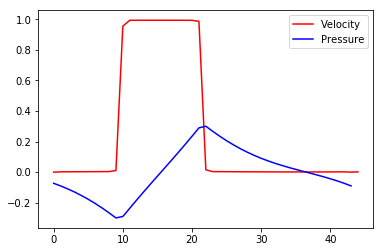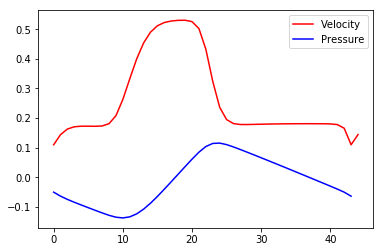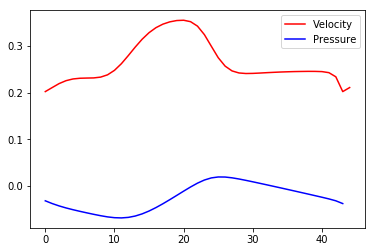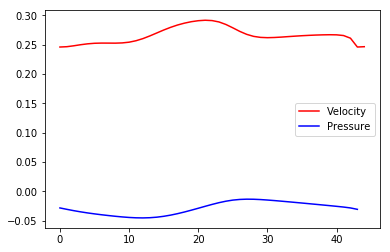taking the derivative of the Navier Stokes Equation with respect to $x$ for reasons which will become clear,

\begin{equation} \frac{\partial^2 u}{\partial x \partial t } + u \frac{\partial^2 u}{\partial x^2} + (\frac{\partial u}{\partial x})^2 = - \frac{\partial^2 P}{\partial x^2} + \mu \frac{\partial^3 u}{\partial x^3} \end{equation} Using the incompressible mass continuity, we can set any derivative of $\frac{\partial u}{\partial x}$ to zero, so,

\begin{equation} \frac{\partial^2 u}{\partial x \partial t } = - \frac{\partial^2 P}{\partial x^2} \end{equation}